# Contact problems of the theory of heat conduction

Problems on the propagation of heat (stationary and non-stationary, for elliptic and parabolic equations, respectively) in the case when the material is thermally inhomogeneous, that is, consists of several parts with different coefficients of thermal conductivity, heat capacityand density. The coefficients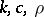entering in the differential equation have discontinuities of the first kind. This leads to problems with weak discontinuities of the solutions, that is, the temperatureis continuous and the derivatives are discontinuous. However, the heat flow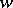is defined to be continuous.

Suppose, for example, that one has a one-dimensional heat equation in,,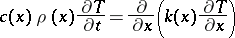(1)

and suppose that at a point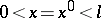the functionshave discontinuities of the first kind,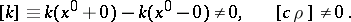Then the temperatureand flow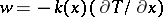must be continuous at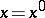(see , ),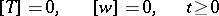(2)

(adjointness conditions). At other points of the interval the temperature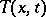must satisfy equation (1) for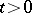, initial conditions for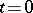, and also boundary conditions for,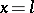,.

In the multi-dimensional case with the parabolic equation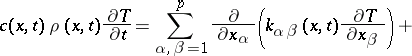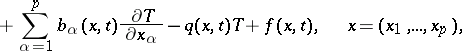one again imposes on the surface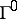of discontinuity of the coefficients the conditions (2) of continuity of the functionand of the flow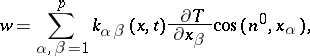where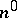is the normal toand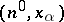is the angle betweenand the direction of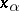(see , ).

In the stationary case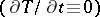, conditions (2) are imposed at the discontinuity. Sometimes more general conservation laws are imposed (see , ). E.g., in the one-dimensional case one considers the following conditions: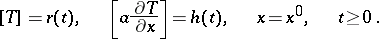Related to the contact problems of the theory of heat conduction is the problem of the propagation of heat in media whose aggregate state can change at a certain value of the temperature(the temperature of phase transition) with emission or absorption of latent heat(the Stefan problem, ). At the unknown boundaryof the phase interface the following conditions are imposed in the one-dimensional case: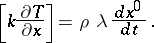There are a large number of contact problems for systems of equations of heat conduction and for equations related to gas dynamics and magneto-hydrodynamics.

How to Cite This Entry:
Contact problems of the theory of heat conduction. Encyclopedia of Mathematics. URL: http://encyclopediaofmath.org/index.php?title=Contact_problems_of_the_theory_of_heat_conduction&oldid=53997
This article was adapted from an original article by I.V. Fryazinov (originator), which appeared in Encyclopedia of Mathematics - ISBN 1402006098. See original article